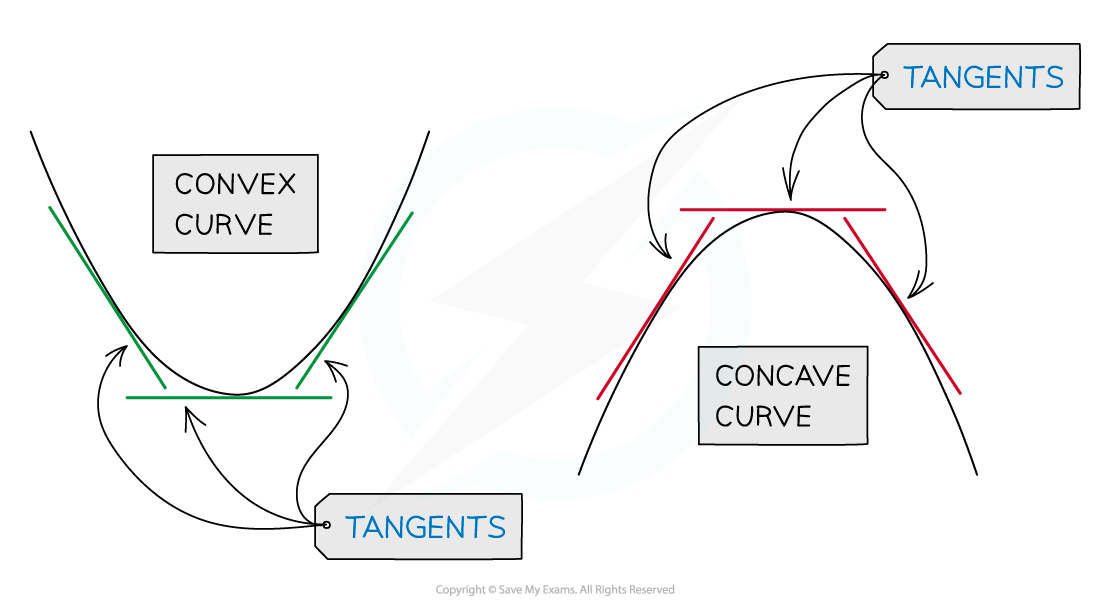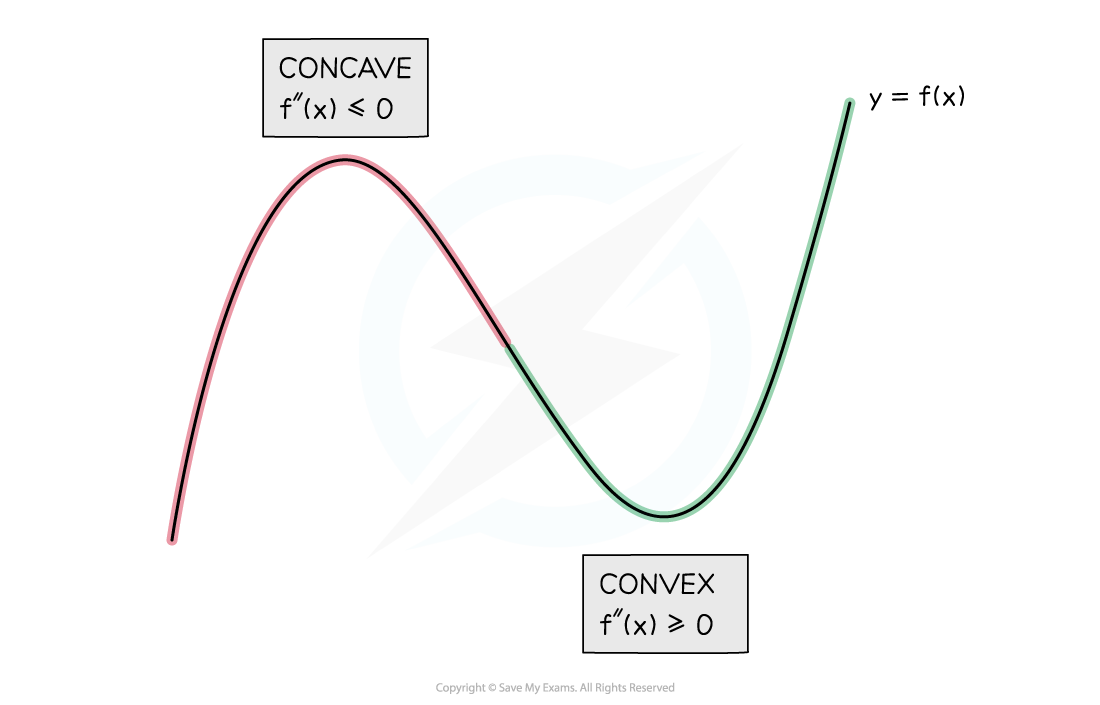# AQA A Level Maths: Pure复习笔记7.4.1 Applications of the Second Derivative

### Applications of the Second Derivative

#### What applications of the second derivative do I need to know?

• The second order derivative (or simply second derivative) is encountered at AS level
• At AS level second derivatives are used to help determine the nature of a stationary point
• At A level you need to be able to use the second derivative to determine if a function is convex or concave on a given interval
• Where a function is convex its graph 'curves up' and its tangent lines lie below the graph
• Where a function is concave its graph 'curves down' and its tangent lines lie above the graph• It's the sign of the second derivative (+ or -) that's important:
• A function f(x) is convex on a given interval if and only if f''(x) ≥ 0 for every value of x in the interval
• A function f(x) is concave on a given interval if and only if f''(x) ≤ 0 for every value of x in the interval#### Exam Tip

• To get full marks in an exam your working needs to include the condition for a function to be convex or concave.

#### Worked Example